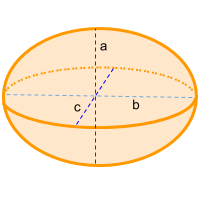Home » Non-polyhedra » Ellipsoid

# Volume of an EllipsoidVolume =
4π/3
×a×b×c

## Volume calculator for an ellipsoid

Enter the height (a), length (b) and width (c) of the ellipsoid to get the volume automatically.

## Description, how many faces, edges and vertices are there in an ellipsoid

The ellipsoid could be seen as a sphere but flattened in its axes or could be seen as an 2-dimensions ellipse which when rotated on its short or long axis ends up forming a 3-dimensional shape, which would be considered an ellipsoid. The ellipsoidhas a single curved face, no edges nor vertices.

## Examples of an ellipsoid

We can find many objects that look like an ellipsoid, examples of ellipsoid shaped objects are: American football, rugby ball, lentils and the most interesting of all, the planet earth; which may seem like a sphere but is actually flatter at the poles, making it an ellipsoid. Can you think of any other examples? leave a comment in the comment section at the bottom of the page.

## Formula for the volume of an ellipsoid

To calculate the volume of an ellipsoidit is necessary to know the height, length and width; then you need to multiply 4/3 by π (Pi = ~ 3.14) and finally multiply this also by the height, length and width. You can also use the online calculator to automatically calculate the volume of the ellipsoid.

Volume =
4π/3
×a×b×c

Share